# Class 11 RD Sharma Solutions – Chapter 6 Graphs of Trigonometric Functions – Exercise 6.2

• Last Updated : 08 May, 2021

### (i) y = cos (x+)

Solution:

To obtain this graph y-0 = cos (x+),

Shifting the origin at, we have

X = x+and Y = y-0

Substituting these values, we get

Y = cos X

First we draw the graph of Y = cos X and shift it byto the left.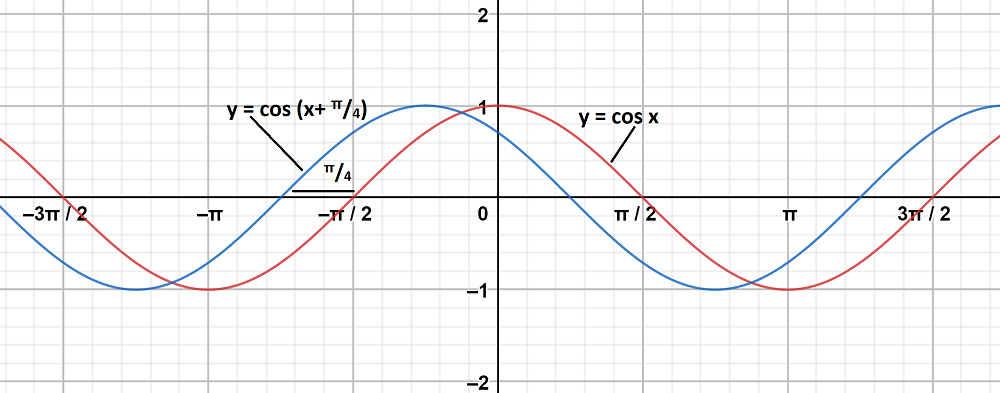### (ii) y = cos (x-)

Solution:

To obtain this graph y-0 = cos (x-),

Shifting the origin at, we have

X =and Y = y-0

Substituting these values, we get

Y = cos X

First we draw the graph of Y = cos X and shift it byto the right.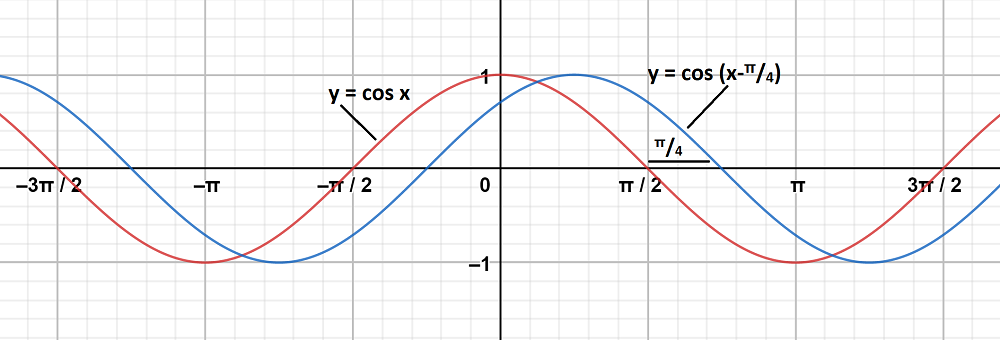### (iii) y =Solution:

To obtain this graph y-0 = 3 cos 2(x-),

Shifting the origin at, we have

X =and Y = y-0

Substituting these values, we get

Y = 3 cos 2X

First we draw the graph of Y = 3 cos 2X and shift it byto the right.

The maximum and minimum values of y are 3 and -3 respectively.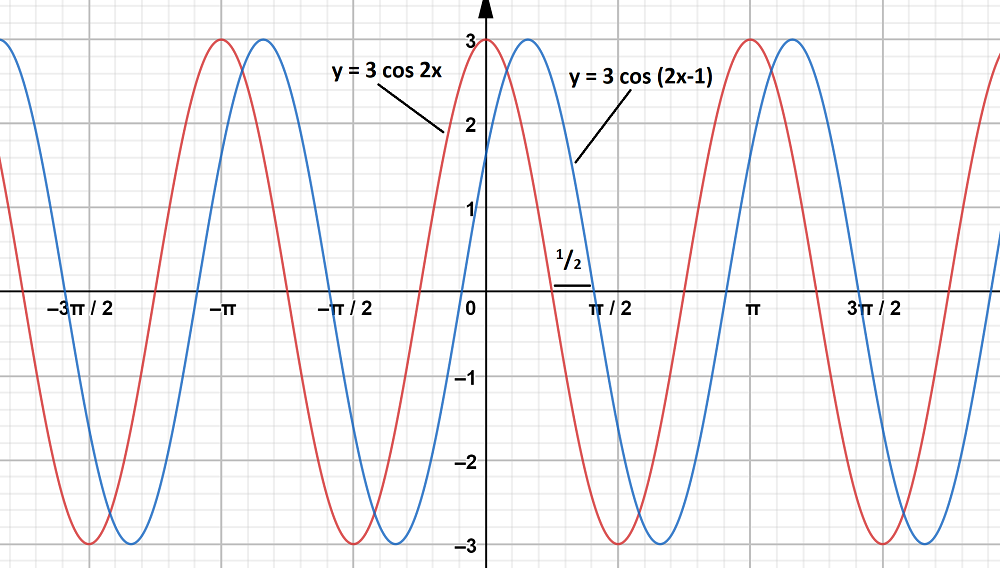### (iv)Solution:

To obtain this graph y-0 =,

Shifting the origin at, we have

X =and Y = y-0

Substituting these values, we get

Y = 2 cos X

First we draw the graph of Y = 2 cos X and shift it byto the right.

The maximum and minimum values of y are 2 and -2 respectively.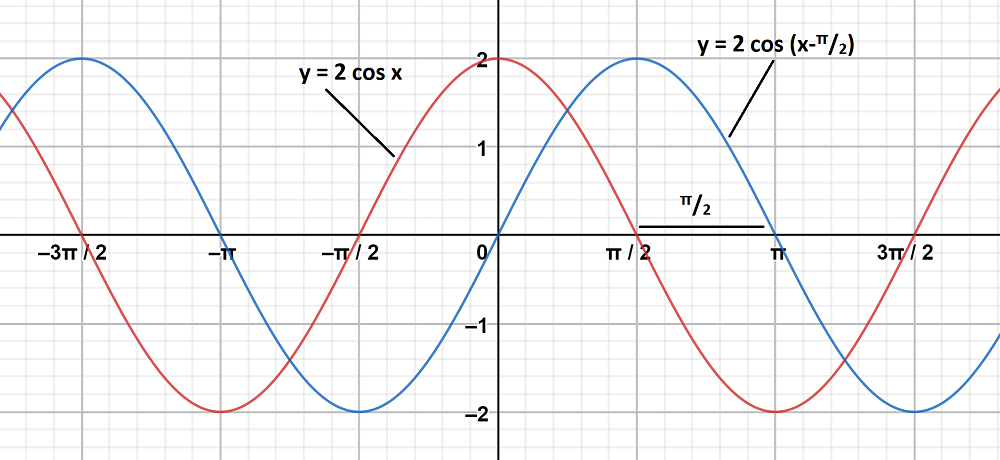### (i) y = cos x, y = cosSolution:

Graph 1:

y = cos x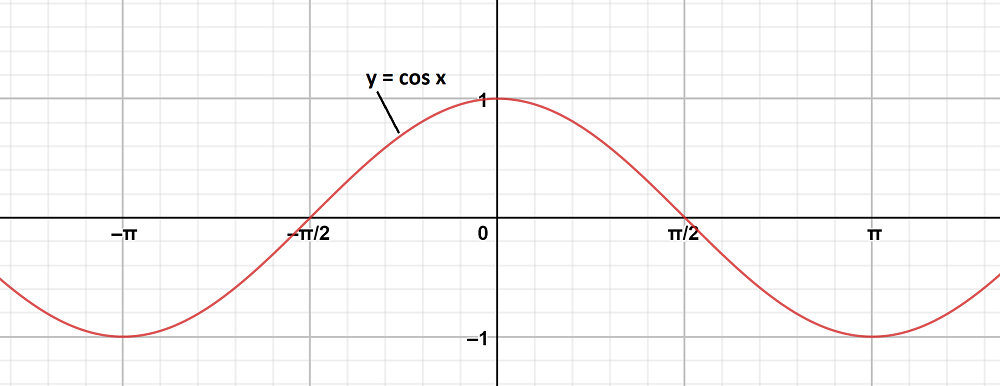Graph 2:

To obtain this graph y-0 = cos,

Shifting the origin at, we have

X =and Y = y-0

Substituting these values, we get

Y = cos X

First we draw the graph of Y = cos X and shift it byto the right.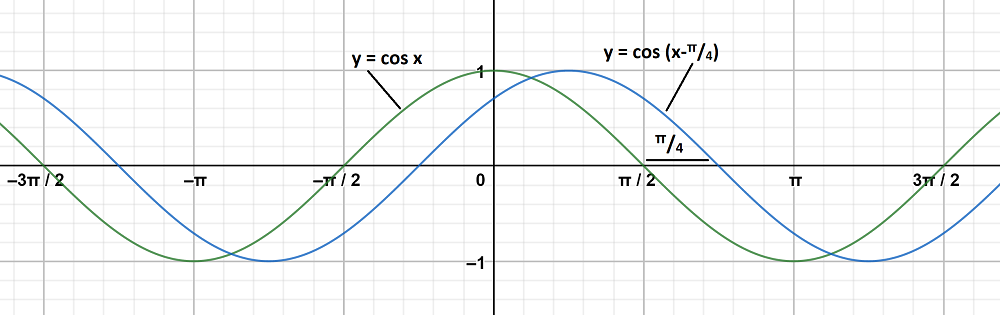The graph y = cos x andare on same axes are as follows: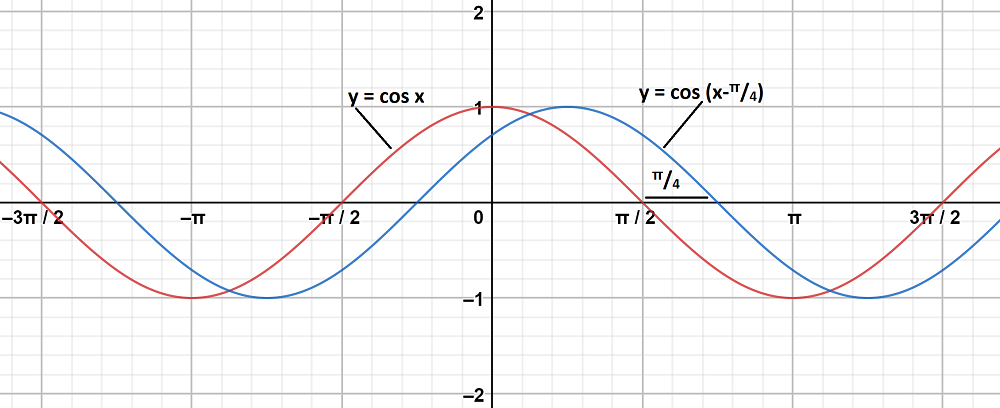### (ii) y = cos 2x, y = cosSolution:

Graph 1:

To obtain this graph y = cos 2x,

First we draw the graph of y =  cos x and then divide the x-coordinates of the points where it crosses x-axis by 2.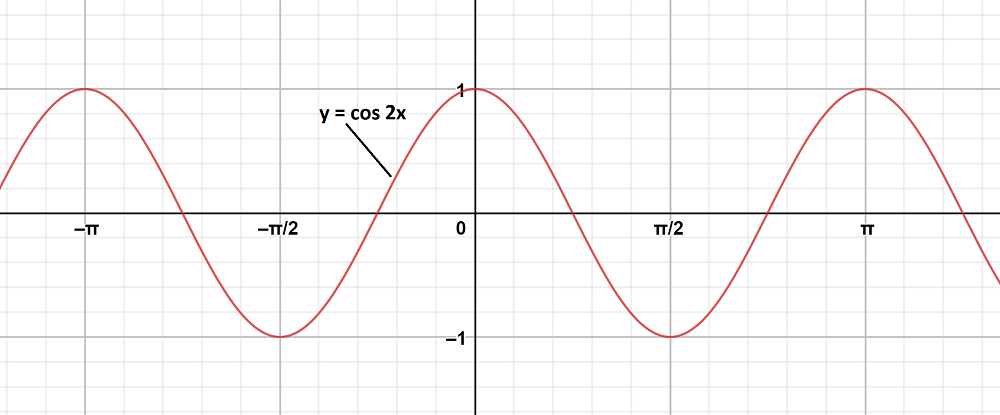Graph 2:

To obtain this graph y-0 = cos,

Shifting the origin at, we have

X =and Y = y-0

Substituting these values, we get

Y = cos 2X

First we draw the graph of Y = cos 2X and shift it byto the right.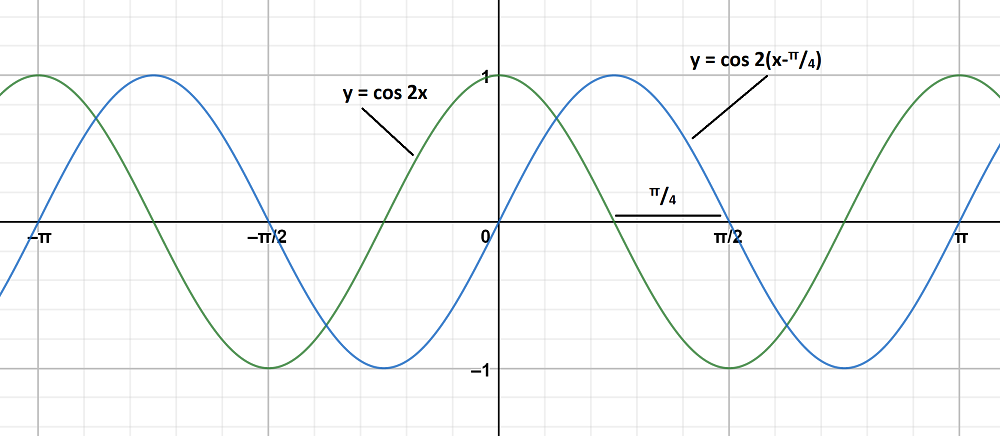The graph y = cos 2x and y = cosare on same axes are as follows: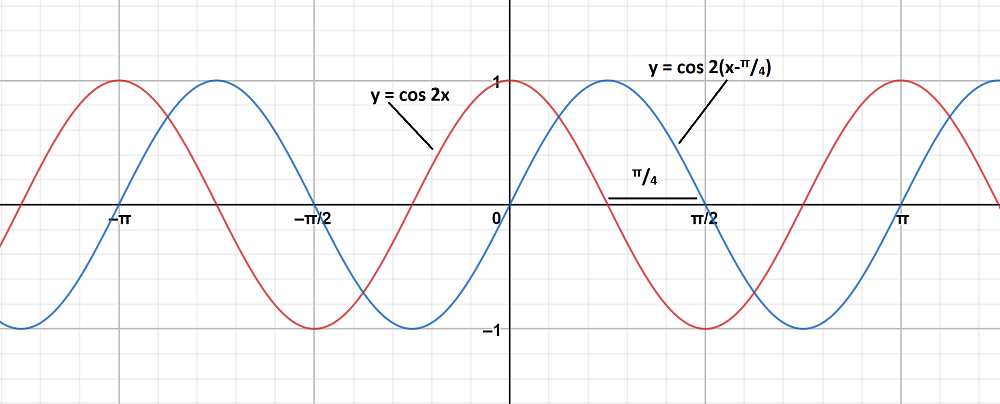### (iii) y = cos x,Solution:

Graph 1:

y = cos x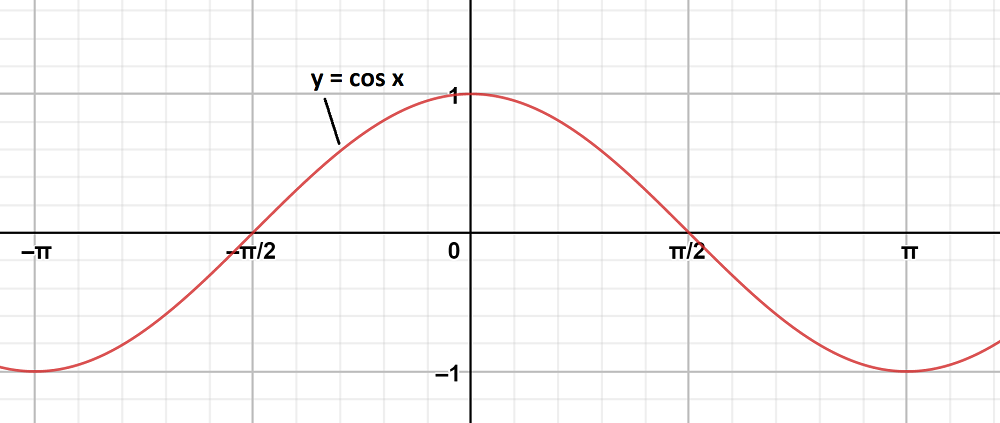Graph 2:

To obtain this graph y =,

First we draw the graph of y =  cos x and then multiply the x-coordinates of the points where it crosses x-axis by 2.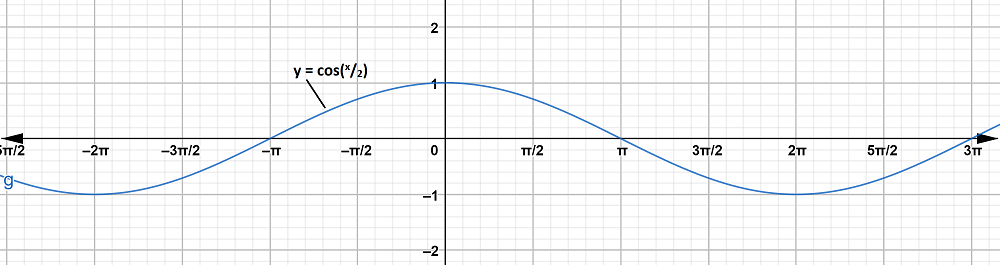The graph y = cos x and y =are on same axes are as follows: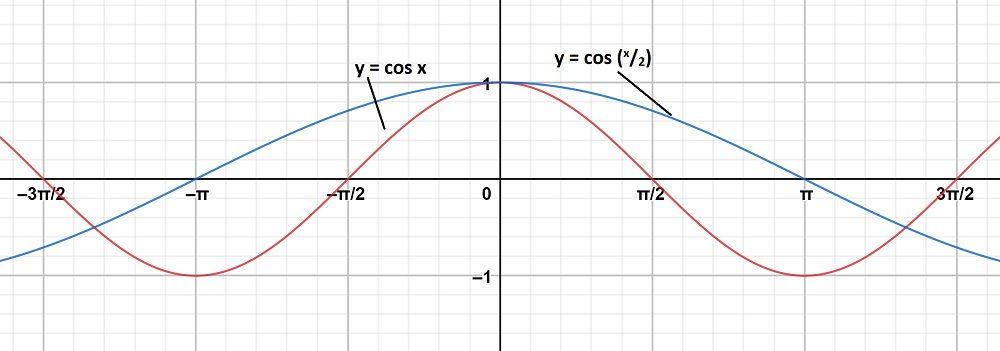My Personal Notes arrow_drop_up